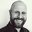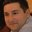Question-and-Answer Resource for the Building Energy Modeling Community
Get started with the Help page

# Converting Annex42 power curve to Normalized power curve for fuel cell CHPI have a fuel cell as part of a model and I'd like to scale it up to better match the heat demand of the dwelling.

The only source I have for the parameters of a power curve are those in the Microcogeneration.idf example file, shown below. This curve is used in the Generator:FuelCell:PowerModule object, and is used in Efficiency Curve Mode, Annex42. This indicates that it's not normalised (unlike with other power curves in EnergyPlus). The documentation suggests that when a study is looking at changing the power output of the fuel cell then we should use mode Normalized.

My question then is what do I need to do to this Curve:Quadratic object to make it into a Normalized curve?

Curve:Quadratic,
FCT Power Curve,         !- Name
0.642388,                !- Coefficient1 Constant
-1.619E-4,               !- Coefficient2 x
2.26007E-8,              !- Coefficient3 x**2
0.0,                     !- Minimum Value of x
10000;                   !- Maximum Value of x


From the documentation:

Field: Efficiency Curve Name

This field contains the name of a Curve:Quadratic input object described elsewhere in the input file. The curve is a function of the net DC electric power,(W), produced by the power module and describes either the electrical efficiency or the how the efficiency is modified depending on the mode selected in the previous field. This quadratic curve is just one part of the entire parametric relation used to describe electrical efficiency. For the Annex42 mode the full relation for efficiency is,where the Curve:Quadratic input object named in this field serves to define the quadratic portion in the first set of brackets. For the Normalized mode the full relation for efficiency is,whereis the nominal electrical efficiency at the rating pointwhich are described in the next two fields. The Normalized mode may be more useful for a simulation study that needs to vary the capacity of the FC.

The nominal values given in the Microgeneration.idf are: nominal power (Pnom) = 3400 Watts, and nominal efficiency (enom) = 0.353.

edit retag close merge delete

Sort by » oldest newest most votedMay I try first :)

Normalised parameters are: 0.5005, -1.2614, 1.7609

I've based them on an assumption that the nominal efficiency has been estimated for FC with no degradation (right part of equation you provided is 1).

Another assumption is that the nominal efficiency is estimated for the nominal power Pnom of 10000 (maximum value of x in the Curve:Quadratic object). This results in enom = 1.283458

We can also define part load ratio PLR as a ratio between Pel and Pnom which gave us following equation

e0 + e1 * Pel + e2 * Pel^2 = enom * (eA + eB * PLR + eC * PLR^2)

e0, e1 and e2 are 0.642388, -1.619E-4, 2.26007E-8 respectively.

Just for ease of creating a table the above equation can be shorten: M = enom * Q where M = e0 + e1 * Pel + e2 * Pel^2 and Q = eA + eB * PLR + eC * PLR^2

Let's add some values to table:

Pel PLR M   Q = M / enom
0   0   0.642   0.501
1000    0.1 0.503   0.392
2000    0.2 0.409   0.319
3000    0.3 0.360   0.281
4000    0.4 0.356   0.278
5000    0.5 0.398   0.310
6000    0.6 0.485   0.378
7000    0.7 0.617   0.480
8000    0.8 0.794   0.618
9000    0.9 1.016   0.792
10000   1   1.283   1.000


The last step is to fit the last column to the quadratic function of PLR which ultimately gives values mentioned at the beginning of the answer. Please redo calculations just in case I made some mistake.

Cheers, IK

more

Thanks Ivan. Sorry, I should have said, Pnom is 3400 Watts and enom is 0.353. I'll try and redo your calculations on that basis and see if the result makes sense, otherwise I'll come back to you.

I think in order to use quadratic equation based on coefficients you provided in the original post you need to use Pnom of 10000. However, if you already have your enom of 0.353 you can recalculated coefficient I mentioned by multiplying them with (1.283458 / 0.353) which results in 1.8198, -4.5864, 6.4025. Your Pnom of 3400W is used to calculate PLR. This should work, hopefully :)

Ok. Well that answer gives the same curve as the one I got from asking the question at Math StackExchange. It seems strange that the efficiency goes up to > 100% at around 9 kW. Perhaps I'm not understanding quite what it's used for.

Ok, If I assume that my starting assumption is wrong (Pnom is just some power at part load operation rather than Pmax) than function coefficients are: 1.8188, -1.5585 and 0.7397. However, in this case P/Pnom varies between 0 (for P=0) and 2.94 (for P=10000). Bare in mind that enom of 0.353 for Pnom of 3400 is calculated by using FCT Power Curve coefficients (which are valid between 0 and 10000).